International Journal of Geomagnetism and Aeronomy
Vol 1, No. 3, August 1999

# Effect of vertical motion of the hydroxyl nightglow layer on the observed variation of rotational temperature

N. N. Pertsev and V. I. Perminov

Institute of Atmospheric Physics, Russian Academy of Sciences, Moscow, Russia

R. P. Lowe and R. DeSerranno

Department of Physics and Astronomy, The University of Western Ontario, London, Canada

### Abstract

Joint spectral observations of the mesopause hydroxyl emissions in the wavelength range 1000-1600 nm were made with a Fourier transform spectrometer of The University of Western Ontario, Canada, and a spectrograph of Institute of Atmospheric Physics, Russia, at Zvenigorod (55.7o N, 36.8o E). The derived data and applied technique of their analysis allow extraction and removal of the effect of temporal changes of the OH emission layer from the obtained rotational temperature data.

### Introduction

During the last three decades the ground-based observations of vibrational-rotational bands in the hydroxyl airglow have widely been used to measure temperature in the region close to the mesopause. As a rule, many researchers in their analyses relate the hydroxyl temperature to the surroundings that at a fixed altitude of about 87 km is consistent with rocket measurements of a peak altitude of the hydroxyl emission rate [Baker and Stair, 1988]. However, current observations of the Wind Imaging Interferometer (WINDII) on board the Upper Atmosphere Research Satellite (UARS) show the remarkable tidal variations of the OH emission peak altitude [Lowe, 1995; Lowe et al., 1996]. This effect can significantly complicate a ground-based observation analysis of temporal variations of the rotational temperature. In the paper by Mulligan and Nallen  we see some discrepancy between observations and model expectations of the tidal characteristics. A possible solution to this problem may be a combined analysis of temporal behavior of the rotational temperature and population ratios of vibrational levels (hereinafter the vibrational ratios). The basic idea is the following. The vibrational ratios are governed by processes of both excitation and deactivation. However, their variations are basically due to changes in the number density of atmospheric species deactivating OH( v ) [Perminov et al., 1997, 1998]. In the case of high vibrational levels these atmospheric species are molecular oxygen and nitrogen. Vibrational deactivation by atomic oxygen decreases with vibrational level and is negligible for the higher levels. Thus a change in the population ratio of the higher levels should co-vary with a change in the number density of the major atmospheric species in the emission layer. Comparing the ground-based measurements of the tidal variations of this vibrational ratio and temperature, it is reasonable to expect their correlation to be close to linear, since the tidal processes are adiabatic. However, this would only hold true if the vertical motion of the OH emission layer occurs in a region where the vertical temperature gradient changes its sign (i.e., around the mesopause). A large amplitude of the vertical excursions of the emission layer would make the correlative function be significantly modulated by the height temperature profile. Correcting this function, we can infer the real characteristics of the tidal variations.

In the present paper we describe briefly the data obtained during a measurement campaign at Zvenigorod. Then some of the results are analyzed to determine the effect of the vertical motion of the hydroxyl emission layer on extracting the semidiurnal tide in the temperature observed. A correlative comparison of the variations of the temperature and population ratio of the vibrational levels 8 to 9 allowed us to make such an analysis. Its importance for the correct evaluation of the semidiurnal tide parameters is also shown.

### Observations

Joint observations by groups from the University of Western Ontario (UWO), Canada, and Institute of Atmospheric Physics (IAP), Russia, were made with two instruments at the Zvenigorod observing site (55.7o N, 36.8o E), Russia, during March 1995. The UWO instrument is a Fourier transform (FT) infrared spectrometer operating in the spectral region 1000-1650 nm, including OH bands of the Dv=2 and Dv=3 sequences. Various versions of this instrument have been used in several studies [Turnbull and Lowe, 1991; Lowe and Turnbull, 1995]. The 10o field-of-view spectrometer was directed at the zenith. One interferogram was produced every 30 s.

The IAP instrument is a grating spectrograph equipped with a three-stage intensified image tube whose output is recorded on photographic film [Potapov et al., 1978]. The photocathode surface of the tube covered simultaneously the spectrum of the OH(4,1) and (9,5) bands in ranging from 1000 to 1060 nm. The duration of each exposure was 6 min. To improve the signal-to-noise ratio, the 10o field of the instrument view was directed toward the north at a 60o zenith angle.

The relative spectral responses of both instruments were determined by calibration with low-brightness sources with a known relative spectral radiance distribution. This permitted the derivation of the rotational temperature and the relative populations of hydroxyl vibrational levels with a precision of 1% to 3%. The rotational temperature and population for the vibrational level 4 (Pop(4)) were determined from the radiance intensities of the P1(2), P1(3), and P1(4) lines in the OH(4,2) and (4,1) bands; the population of vibrational level 8 (Pop(8)), from those of the P1(3) and P1(4) lines in the (8,5) band; and the population of vibrational level 9 (Pop(9)), from those of the P1(2) and P1(3) lines in the (9,5) band. All of these lines are relatively free of absorption by atmospheric gases and not blended with lines of bands of other sequences, which lets us avoid this potential source of errors. In order to calculate the populations, the radiative transition probabilities of Turnbull and Lowe  were used.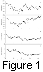Joint observations were made on four nights, but the observations on three of them were not of adequate quality because of the appearance of thin clouds and combined with some instrumental difficulties. Only one night, March 12-13, 1995, combined clear weather with a long data series. The data from this night were used for further analysis. Measurements of OH band spectra during the night were carried out from 2016 to 0434 LT. Figure 1 presents the temporal behavior of the rotational temperature and ratios between populations of vibrational levels 4 and 8 (observations with the FT spectrometer) and between populations of vibrational levels 4 and 9 (observations with the spectrograph). Each point is the mean value of the ratio or temperature for 6 min. As can readily be seen, the general character of the variations from the two instruments are the same. The higher scatter of the temperature values from the OH(4,1) band are likely due to a lower signal-to-noise ratio in the spectrographic measurements in comparison with those from the FT spectrometer. Nevertheless, the mean temperatures from the two instruments (204 K and 203 K, respectively) are in good agreement.

The observations allowed us to infer rotational temperatures from the (8,5) and (9,5) bands as well. However, in our analysis we do not use them, since the precision of their determination was significantly lower in comparison with the temperatures inferred from the (4,1) and (4,2) bands. It would be desirable to search for differences in rotational temperature as a function of vibrational level, since the height profile of the emission may be slightly different as a result of quenching. Unfortunately, this is not feasible with the present data since the precision of the temperatures obtained from the bands involving higher vibrational levels is significantly less, as was noted above. In addition, Turnbull and Lowe  have shown that the absolute value of the rotational temperature obtained depends significantly on the choice of transition probabilities used, particularly for high vibrational levels. Hence we have restricted our discussion to the (4,1) and (4,2) bands. In fact, the differences in temperature found for v = 4, 8 and 9 were sufficiently small to imply that only small difference in height profile could be present.

### Analysis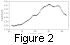In the present analysis, we used the variation of the population ratio of the eighth to the ninth vibrational level, z= Pop(8)/Pop(9). As was mentioned above, such a vibrational ratio should co-vary with the number densities of the major atmospheric species in the hydroxyl emission layer. Thus z may play the role of a vertical coordinate. The time series of z(t) ( t denotes time) was obtained as follows. Since the observations of Pop(8) and Pop(9) were made with different instruments looking in different directions, it was first necessary to refer both sets of data to the same direction, using the fact that both instruments observed Pop(4) as a guide. The underlying assumption of the analysis used was that the long time scale variations observed by the two instruments, corresponding to larger spatial scales, should be similar but with the possibility of phase shifts. The process followed was to filter both the time series of Pop(4)/Pop(9) from the northward looking instrument and Pop(4)/Pop(8) from the zenith looking instrument to eliminate variations with periods of less than 2 hours, since such rapid fluctuations were more likely to be different in the two directions. The resulting time series were then approximated by a series of sinusoids with the same periods (from 2 to 12 hours) by least squares fitting and correlative analysis using the technique developed by Vitinsky et al. . It was found, as expected, that the phases of the components with periods of greater than 4-hours were in good agreement but that the phases of those components with periods from 2 to 4 hours differed slightly between the two time series. Accordingly, to adjust the two time series to a common direction of look (zenith), the phase of the components with 2 to 4 hours for the time series Pop(4)/Pop(9) were adjusted to agree with those of the Pop(4)/Pop(9). Finally, the time series of z(t) = Pop(8)/Pop(9) was found by simple division of the two time series. The result is shown in Figure 2.

##### Variations of rotational temperature.

It is important to emphasize that the hydroxyl rotational temperature does not relate to either a constant altitude or to a constant density. For investigations of thermodynamic properties of atmospheric oscillations it would be convenient to know the temporal variation of temperature on the surface of a constant neutral density. Any variations in the shape of the vertical profile of the OH emission layer would be accompanied by a change in the population ratio, so that a measurement of rotational temperature is carried out in changing conditions. Thus it is desirable to remove an effect of the changing conditions from the rotational temperature series. To provide such an analysis, we make the assumption that the rotational temperature T is a function of two arguments:(1)

Here we temporarily ignore the time dependence of z = Pop(8)/Pop(9) and regard it as an independent variable. Below we made an effort to divide T(z, t) into two parts:(2)

Such type of separation would be much more sophisticated if z varied in time monotonically. Fortunately, for that observation, the behavior of zt) was fairly close to semidiurnal sinusoid (see Figure 2), and a large portion of temperature data referred to the different time moments and to the same  z. It simplified the analysis substantially.

The first part of (2), Ta(z), describes the variation of temperature caused by excursions and redistributions of the measuring probe (i.e., the OH emission layer) in the neutral density field. The second one gives the temporal variation of temperature on an isostere (i.e., on the surface of the constant neutral density).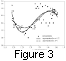The Ta(z) may be found (see Figure 3) by plotting the measurements of the rotational temperature from the OH(4,2) band versus z and then by approximating the aggregate of the points (z, T) by a four term polynomial expression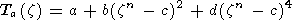(3)

using the least squares fit. The fifth parameter n, being external, was set to investigate a sensitivity of results to the arbitrariness of the approximation (3). In Figure 3 and below the results for n=1/2 and n=1 are shown.After obtaining Ta(z) we can find DT(t). The later curve, as well as T1(t) = Ta(z (t)) -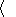T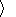and T0(t) =T (z(t), t) -T, is plotted in Figure 4. The value of the average temperatureTis 203 K. According to Figure 4, there are significant differences in the interpretation of the three functions T0(t), T1(t), and DT(t). All of these of course depend on the validity of the approximation of Ta(z). In this approach, T1 (t) is an estimation of the temperature variation caused by motions and distortions of the hydroxyl emission layer in a time-independent background temperature field, whereas DT(t) estimates the temperature variations that would occur on an isostere; T0(t) is influenced by both processes. It is worthwhile to examine the effect of the differences in amplitude and phase between the three curves on the semidiurnal tide.

##### Semidiurnal tide.

Figure 2 demonstrates a distinct pattern of the semidiurnal variation in  z. Semidiurnal tide parameters derived from T(t) and DT(t) may differ substantially, depending on the vertical profile of temperature. Extracting the 12-hour wave from T(z, t), we derive the wave caused by both a change of the vertical coordinate of the OH emission peak and a change of temperature on an isostere. To derive the 12-hour wave from DT(t) is much more physically reasonable. In this case we extract the temporal variation of temperature on an isostere only. The results of the least mean square fitting of the curves T0(t), T1(t), and DT(t), shown in Figure 4, to the 12-hour sinusoid are given in Table 1. Obviously, both the amplitude and phase for semidiurnal tide were changed substantially after removing Ta(z (t)) from the temperature series. After the reduction of the OH emission layer to a temporally uniform situation in the neutral density field, the amplitude decreased from 8 K to 6 K, and the phase changed from 5 hours to 6 hours. The choice of one or another approximation for Ta(z(t)) seems to be of less importance: the two distinctly different approximations (for n=1/2 and n=1 ; see Figure 4) give a difference which is negligible in comparison with the typical error of the fitting procedure, shown in Table 1 for the amplitude and phase of the semidiurnal tide.

The difference between the semidiurnal parameters for T0 (t) and DT(t) must depend strongly on the vertical profile of temperature. That is why we may refer some part of the strong spatial and temporal variability of the semidiurnal tide parameters, measured by OH rotational temperature, to the variability of the temperature vertical profile.

### Conclusions

The data of the hydroxyl nightglow observations were used to extract the effect of the vertical motions of the OH emission layer from its rotational temperature variations. Such changes of the OH emission layer in the neutral density field may be retraced using temporal variations of the vibrational population ratio Pop(8)/Pop(9). Regarding the OH emission layer as a measuring probe for temperature of the mesopause region, one should reduce the results to a situation where the layer vertical profile is invariant with respect to some vertical coordinate. Such a procedure has been shown to be best justified if the rotational temperature behaves non-monotonically in time. It leads to the possibility of estimating the temperature variation which is independent on the behavior of the OH emission layer.

Based on the data from one night, such a temperature curve was obtained. This effect also proves to be important in the derivation of the parameters of the semidiurnal tide. Some part of the strong spatial and temporal variability of the semidiurnal tide parameters, measured by OH rotational temperature, seems to be caused by a variability of the temperature vertical profile.

#### Acknowledgments

Partial support for this study was provided by a grant from the Natural Sciences and Engineering Research Council of Canada. The Centre for Research in Earth and Space Technology is an Ontario Centre-of-Excellence. The authors are grateful to N. N. Shefov and A. I. Semenov for their encouragement of this investigation.

### References

Baker, D. J., and A. T. Stair, Rocket measurements of the altitude distributions of the hydroxyl airglow, Phys. Scripta, 37, 611-622, 1988.

Lowe, R. P., Correlation of WINDII/UARS airglow emission heights with ground-based rotational temperature, 22nd European Meeting on Atmospheric Studies by Optical Methods (Nurmijarvi, Finland, August 28 - September 1, 1995), Abstracts, G-2, 1995.

Lowe, R. P., and D. N. Turnbull, Comparison of ALOHA-93, ANLC-93 and ALOHA-90 observations of the hydroxyl rotational temperature and gravity wave activity, Geophys. Res. Lett., 22, 2813-2816, 1995.

Lowe, R. P., L. M. LeBlanc, and K. L. Gilbert, WINDII/UARS observation of twilight behavior of the hydroxyl airglow, at mid-latitude equinox, J. Atmos. Terr. Phys., 58, 1863-1869, 1996.

Mulligan, F. J., and J. J. Nallen, Amplitudes and phases of the diurnal and semidiurnal tide observed in mesospheric OH(3,1) emission over Maynooth, Ireland, Proc. of SPIE, 3237, 154, 1996.

Perminov, V. I., et al., OH( v=9 ) radical deactivation by molecular oxygen from the nightglow observations, in Abstracts for the 8-th Scientific Assembly of IAGA with ICMA and STP Symposia, p. 162, Reclam and Katalogtryck, Uppsala, 1997.

Perminov, V. I., A. I. Semenov, and N. N. Shefov, Deactivation of vibrational levels of hydroxyl molecule by atomic and molecular oxygen in the mesopause region, Geomagn. Aeron., 38 (6), 100, 1998.

Potapov, B. P., et al., Internal gravity waves near the mesopause, II, Instruments and optical methods of measurements, in Aurora and Airglow, 26, vol. 26, pp. 30-65, Soviet Radio, Moscow, 1978.

Turnbull, D. N., and R. P. Lowe, New hydroxyl transition probabilities and their importance in airglow studies, Planet. Space Sci., 37, 723-738, 1989.

Turnbull, D. N., and R. P. Lowe, Temporal variations in the hydroxyl nightglow observed during ALOHA-90, Geophys. Res. Lett., 18, 1345-1348, 1991.

Vitinsky, Yu. I., M. Kopetsky, and G. V. Kuklin, Statistics of Sunspot Activity, 296 pp., Nauka, Moscow, 1989.

Load files for printing and local use. This document was generated by TeXWeb (Win32, v.2.0) on September 23, 1999.